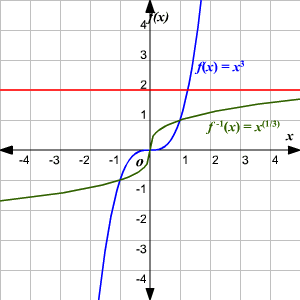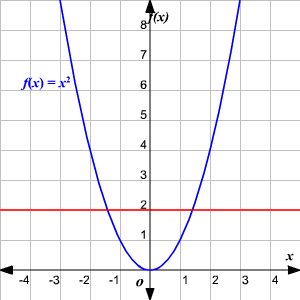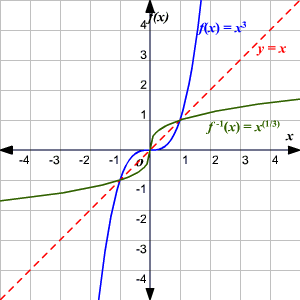# One-to-One Functions

A function $f$ is $1$ -to- $1$ if no two elements in the domain of $f$ correspond to the same element in the range of $f$ .  In other words, each $x$ in the domain has exactly one image in the range.  And, no $y$ in the range is the image of more than one $x$ in the domain.

If the graph of a function $f$ is known, it is easy to determine if the function is $1$ -to- $1$ .  Use the Horizontal Line Test.  If no horizontal line intersects the graph of the function $f$ in more than one point, then the function is $1$ -to- $1$ .A function $f$ has an inverse ${f}^{-1}$ (read $f$ inverse) if and only if the function is $1$ -to- $1$ .

## Properties of a $1$ -to- $1$ Function:

1) The domain of $f$ equals the range of $f$ –1 and the range of $f$ equals the domain of ${f}^{-1}$ .

2) ${f}^{-1}\left(f\left(x\right)\right)=x$ for every $x$ in the domain of $f$ and $f\left({f}^{-1}\left(x\right)\right)=x$ for every $x$ in the domain of $f$ –1 .

3)  The graph of a function and the graph of its inverse are symmetric with respect to the line $y=x$ .